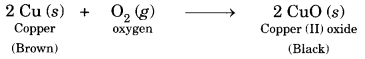# Bihar Board Class 10 Science Solutions Chapter 1 Chemical Reactions and Equations

BSEB Bihar Board Class 10 Science Solutions Chapter 1 Chemical Reactions and Equations Textbook Questions and Answers.

## BSEB Bihar Board Class 10 Science Solutions Chapter 1 Chemical Reactions and Equations

### Bihar Board Class 10 Science Chapter 1 Chemical Reactions and Equations InText Questions and Answers

In-text Questions (Page 6)

Question 1.
Why should a magnesium ribbon be cleaned before burning in air?
Magnesium being a reactive metal combines with oxygen of air to form a layer of magnesium oxide on its surface. Hence, it should be cleaned with a sand paper before burning to remove the oxide layer formed on its surface.

Question 2.
Write the balanced equations for the following chemical reactions:
(i) Hydrogen + Chlorine → Hydrogen chloride
(ii) Barium chloride + Aluminium sulphate → Barium sulphate + Aluminium chloride
(iii) Sodium + Water → Sodium hydroxide + Hydrogen
(i) H2(g) + Cl2(g) → 2HCl(g)
(ii) 3BaCl2(aq) + Al2(SO4)3(aq) → 3BaSO4(s) + 2AlCl3(aq)
(iii) 2Na(s) + 2H2O(s) → 2NaOH(aq) + H2(g)

Question 3.
Write a balanced chemical equation with state symbols for the following reactions:
(i) Solutions of barium chloride and sodium sulphate in water react to give insoluble barium sulphate and the solution of sodium chloride.
(ii) Sodium hydroxide solution (in water) reacts with hydrochloric acid solution (in water) to produce sodium chloride solution and water.
The symbol equations in balanced form for the reactions are:
(i) BaCl2(aq) + Na2SO4(aq) → BaSO4(s) + 2NaCl(aq)
(ii) NaOH(aq) + HCl(aq) → NaCl(aq) + H2O(l)

In-text Questions (Page 10)

Question 1.
A solution of a substance ‘X’ is used for whitewashing.
(i) Name the substance ‘X’ and write its formula.
(ii) Write the reaction of the substance ‘X’ named in (i) above with water.
(i) The substance X’ used for whitewashing is quick lime (calcium oxide). The formula is CaO.
(ii) When quick lime is mixed with water, the following reaction takes place.The white suspension of slaked lime is applied on the walls. It combines with carbon dioxide of the air forming a thin shining layer of calcium carbonate.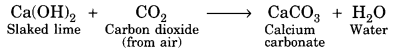Question 2.
Why is the amount of gas collected in one of the test tubes in Activity 1.7 double of the amount collected in the other? Name this gas.
On electrolysis, water decomposes according to the reaction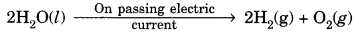Thus water decomposes to give hydrogen and oxygen in the ratio of 2 : 1 by volume. The double volume of the gas collected is hydrogen.

In-text Questions (Page 13)

Question 1.
Why does the colour of copper sulphate solution change when an iron nail is dipped in it?
It is because a displacement reaction takes place. Iron displaces copper from copper sulphate solution forming green colour solution of FeSO4 and reddish brown copper metal gets deposited.
Fe(s) + CuSO4(aq) → FeSO4(aq) + Cu(s)

Question 2.
Give an example of a double displacement reaction other than the one given in Activity 1.10.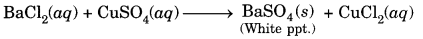Question 3.
Identify the substances that are oxidised and the substances that are reduced in the following reactions.
(i) 4Na(s) + O2(g) → 2Na2O(s)
(ii) CuO(s) + H2(g) → Cu(s) + H2O(l)
(i) In this reaction, sodium (Na) is oxidised to sodium oxide (Na2O). This means that oxygen (O2) has been reduced.
(ii) In this reaction, hydrogen (H2) is oxidised to water (H2O) while copper (II) oxide (CuO) is reduced to copper (Cu).

### Bihar Board Class 10 Science Chapter 1 Chemical Reactions and Equations Textbook Questions and Answers

Question 1.
Which of the statements about the reaction below are incorrect?
2PbO(s) + C(s) → 2Pb(s) + CO2(g)
(b) Carbon dioxide is getting oxidised.
(c) Carbon is getting oxidised.
(d) Lead oxide is getting redused.
(i) (a) and (b)
(ii) (a) and (c)
(iii) (a), (b) and (c)
(iv) All
(i) (a) and (b)

Question 2.
Fe2O3 + 2Al → Al2O3 + 2Fe
The above reaction is an example of a
(a) combination reaction.
(b) double displacement reaction
(c) decomposition reaction.
(d) displacement reaction.
(d) displacement reaction.

Question 3.
What happens when dilute hydrochloric acid is added to iron fillings? Tick the correct answer.
(a) Hydrogen gas and iron chloride are produced.
(b) Chlorine gas and iron hydroxide are produced.
(c) No reaction takes place.
(d) Iron salt and water are produced.
(a) Hydrogen gas and iron chloride are produced.

Question 4.
What is a balanced chemical equation? Why should chemical equations be balanced?
Balanced chemical equation means total number of atoms of each element should be equal on both sides of the reaction. The reaction should be balanced because matter can neither be created nor be destroyed. The total mass of reactants should be equal to total mass of products according to the law of conservation of mass.

Question 5.
Translate the following statements into chemical equations and then balance them.
(a) Hydrogen gas combines with nitrogen to form ammonia.
(b) Hydrogen sulphide gas burns in air to give water and sulphur dioxide.
(c) Barium chloride reacts with aluminium sulphate to give aluminium chloride and a precipitate of barium sulphate.
(d) Potassium metal reacts with water to give potassium hydroxide and hydrogen gas.
(a) 3H2 + N2 → 2NH3 (Combination of reaction)
(b) 2H2S + 3O2 → 2H2O + 2SO2 (Redox Reaction)
(c) 3BaCl2 + Al2(SO4)3 → 2AlCl3 + 3BaSO4(s) (Double displacement reaction)
(d) 2K + 2H2O → 2KOH + H2 (Displacement reaction)

Question 6.
Balance the following chemical equations.
(a) HNO3 + Ca(OH)2 → Ca(NO3)2 + H2O
(b) NaOH + H2SO4 → Na2SO4 + 2H2O
(c) NaCl + AgNO3 → AgCl + NaNO3
(d) BaCl2 + H2SO4 → BaSO4 + 2HCl
(a) 2HNO3 + Ca(OH)2 → Ca(NO3)2 + 2H2O
(b) 2NaOH + H2SO4 → Na2SO4 + 2H2O
(c) NaCl + AgNO3 → AgCl + NaNO3
(d) BaCl2 + H2SO4 → BaSO4 + 2HCl

Question 7.
Write the balanced chemical equations for the following reactions.
(a) Calcium hydroxide + Carbon dioxide → Calcium carbonate + Water
(b) Zinc + Silver nitrate → Zinc nitrate + Silver
(c) Aluminium + Copper chloride → Aluminium chloride + Copper
(d) Barium chloride + Potassium sulphate → Barium sulphate + Potassium chloride
(a) Ca(OH)2(aq) + CO2(g) → CaCO3(s) + H2O(l)
(b) Zn(s) + 2AgNO3(aq) → Zn(NO3)2(aq) + 2Ag(s)
(c) 2Al(s) + 3CuCl2(ag) → 2AlCl3(aq) + 3Cu(s)
(d) BaCl2(aq) + K2SO4(aq) → BaSO4(s) + 2KCl(aq)

Question 8.
Write the balanced chemical equation for the following and identify the type of reaction in each case.
(a) Potassium bromide(ag) + Barium iodide(ag) → Potassium iodide(aq) + Barium bromide(s)
(b) Zinc carbonate(s) → Zinc oxide(s) + Carbon dioxide(g)
(c) Hydrogen(g) + Chlorine(g) → Hydrogen chloride(g)
(d) Magnesium(s) + Hydrochloric acid(aq) → Magnesium chloride(aq) + Hydrogen(g)
(a) 2KBr(ag) + BaI2(aq) → 2KI(aq) + BaBr2(aq)
The reaction is a double displacement reaction.
(b) ZnCO3(s) → ZnO(s) + CO2(g)
The reaction is a decomposition reaction.
(c) H2(g) + Cl2(g) → 2HCl(g)
The reaction is known as combination reaction.
(d) Mg(s) + 2HCl(aq) → MgCl2(aq) + H2(g)
The reaction is a displacement reaction.

Question 9.
What does one mean by exothermic and endothermic reactions? Give examples.
Exothermic reactions: Those reactions which proceed with the evolution of heat energy are called exothermic reaction. For example, burning of fuels, respiration.
Endothermic reactions: Those reactions which proceed with the absorption of heat energy are called endothermic reaction. For example, decomposition of calcium carbonate using heat energy.

Question 10.
Why is respiration considered an exothermic reaction? Explain.
Rice, potatoes, bread, etc. that we eat contain starch. On digestion, it breaks down into glucose. The air we breathe in during respiration oxidises glucose into carbon dioxide and water with the liberation of heat which provides our body with the required energy. Hence, respiration is considered as an exothermic reaction.

Question 11.
Why are decomposition reactions called the opposite of combination reactions? Write equations for these reactions.
Decomposition reactions are called opposite of combination reactions because in a decomposition reaction, a single compound breaks down to form two or more simple substances under the effect of heat, light or electric current, whereas in a combination reaction two or more substances combine to form a single product.
For example,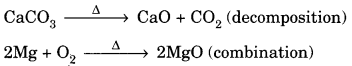Question 12.
Write one equation each for decomposition reactions where energy is supplied in the form of heat, light or electricity.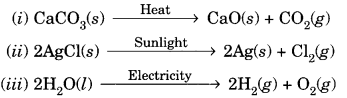Question 13.
What is the difference between displacement and double displacement reactions? Write equations for these reactions.
In a single displacement reaction, a more reactive metal displaces a less reactive metal from its salt solution. For example,
Fe + CuSO4(ag) → FeSO4(aq) + Cu
Iron being more reactive than copper displaces copper from its salt solution, while in a double displacement reaction the exchange of ions takes place between the reactants. For example, on mixing aqueous solutions of barium chloride and sodium sulphate, barium ions and sodium ions exchange their position.
BaCl2(aq) + Na2SO4(aq) → BaSO4(s) + 2NaCl(ag)

Question 14.
In the refining of silver, the recovery of silver from silver nitrate solution involved displacement by copper metal. Write down the reaction involved.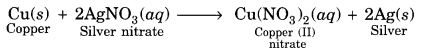Question 15.
What do you mean by a precipitation reaction? Explain by giving examples.
The reaction in which two compounds in their aqueous state react to form an insoluble substance is called precipitation reaction. A precipitate is generally obtained when aqueous solutions of two substances are mixed together. For example, mixing of aqueous solutions of sodium chloride and silver nitrate forms an insoluble precipitate of silver chloride.
NaCl(aq) + AgNO3(ag) → AgCl(s) + NaNO3(aq)

Question 16.
Explain the following in terms of gain or loss of oxygen with two examples each.
(a) Oxidation (b) Reduction
(a) Oxidation. It is defined as a process which involves gain of oxygen. For example,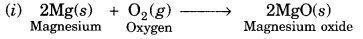Here, Mg has gained oxygen to form MgO. Hence, Mg has been oxidised to MgO.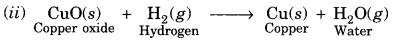Here, H2 has gained oxygen to form H2O. Hence, H2 has been oxidised to H2O.

(b) Reduction. It is defined as a process which involves loss of oxygen. For example,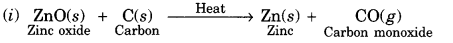Here, ZnO has lost oxygen to form Zn. Hence, ZnO has been reduced to Zn.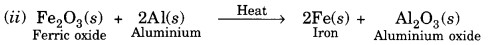Here, Fe2O3 has lost oxygen to form Fe. Hence, Fe2O3 has been reduced to Fe.

Question 17.
A shiny brown coloured element ‘X’ on heating in air becomes black in colour. Name the element ‘X’ and the black coloured compound formed.
The element ‘X’ is copper and the black coloured compound is copper (II) oxide also known as cupric oxide.
$$\underset{(\mathrm{X})}{2 \mathrm{Cu}}+\mathrm{O}_{2} \stackrel{\text { Heat }}{\longrightarrow} \underset{\text { (Black })}{2 \mathrm{CuO}}$$

Question 18.
Why do we apply paint on iron articles?
Paint covers the surface of the iron articles. Hence, moist air cannot come directly in contact with iron. As a result, it cannot attack iron and prevents rusting.

Question 19.
Oil and fat containing food items are flushed with nitrogen. Why?
Oil and fat containing food items are generally flushed with nitrogen to prevent the oxidation of oil and fats. Nitrogen being an inert gas does not react with oils and fats, prevents the oxidation of oil and fats and thus, they last longer without turning rancid.

Question 20.
Explain the following terms with example each:
(а) Corrosion (b) Rancidity
(a) Corrosion: It is a process in which some metal react with oxygen and moisture present in atmosphere to form surface compounds, e.g., iron reacts with oxygen in presence of moisture to form rust, Fe2O3.xH2O (hydrated iron (III) oxide).

(b) Rancidity: It is a process in which food materials get spoilt due to oxidation. It leads to a change in the taste and smell of food materials, e.g., butter gets spoiled due to oxidation at room temperature if kept for a long time. It becomes sour in taste and gives a foul smell also.

### Bihar Board Class 10 Science Chapter 1 Chemical Reactions and Equations Textbook Activities

Activity 1.1 (Textbook Page 1)

Observation: Magnesium ribbon burns with a dazzling white light and change into a white powder of magnesium oxide.
Conclusion: Magnesium burns in air to combine with oxygen and form magnesium oxide.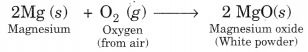Activity 1.2 (Textbook Page 2)

Observation: A yellow solid (precipitate) is formed.
Conclusion: Lead nitrate solution reacts with potassium iodide solution to form a yellow precipitate of lead iodide.
Pb(NO3)2(aq) + 2KI (aq) → PbI2(s) + 2KNO3

Activity 1.3 (Textbook Page 2)

Observation: Bubbles of hydrogen gas are observed to rise briskly from the surface of zinc pieces. The gas evolved can be tested by bringing a lighted candle. It is found to burn with a pop sound.
Conclusion: Zinc react with sulphuric acid or hydrochloric acid with the evolution of hydrogen gas and heat (because reaction is exothermic).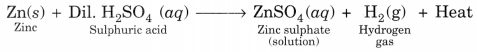Activity 1.4 (Textbook Page 6)

Observation: A vigorous reaction is found to occur and the beaker is found to become very hot since the reaction is exothermic.
Conclusion: Calcium oxide(CaO) reacts with water to form calcium hydroxide (slaked lime) and this reaction is highly exothermic.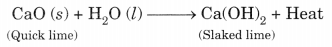Activity 1.5 (Textbook Page 8)

Observation: Green coloured ferrous sulphate crystals (FeSO4. 7H2O) on heating first changes to yellow colour by losing water of crystallisation to form FeSO4, which on further heating gives a reddish brown residue along with evolution of sulphur dioxide(SO2) and sulphur trioxide(SO3) gases.
Conclusion: The reddish brown residue is of ferric oxide.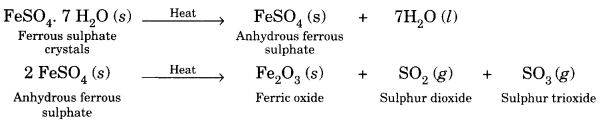Activity 1.6 (Textbook Page 8)

Observation: Brown fumes of nitrogen dioxide (NO2) are evolved and a yellow residue is left in the test tube. Conclusion: Solid lead nitrate decomposes on heating to give out brown fumes of NO2 and a yellow residue of lead (II) oxide.

Activity 1.7 (Textbook Page 9)

Observation: The volume of gas collected over the cathode is double than that collected over the anode. The gas with double volume burns with a pop sound when a lighted splinter is brought near it. Therefore, this gas is hydrogen. The other gas supports burning since it rekindles a glowing splinter. Hence, this gas is oxygen. Conclusion: Acidified water undergoes electrolysis producing H2 and O2 gases in the ratio of 2 : 1 by volume
$$2 \mathrm{H}_{2} \mathrm{O}(l) \stackrel{\text { Electrolysis }}{\longrightarrow} 2 \mathrm{H}_{2}(g)+\mathrm{O}_{2}(g)$$

Activity 1.8 (Textbook Page 9)

Observation: White crystals of silver chloride turn grey in the presence of sunlight.
Conclusion: Silver chloride decomposes into grey silver and chlorine in the presence of sunlight.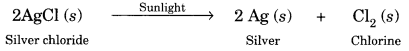Activity 1.9 (Textbook Page 10)

Observation: The iron nails become brownish in colour and the blue colour of copper sulphate solution fades and changes to light green colour in test tube B.
Conclusion: Iron displaces copper from copper sulphate solution forming iron (II) sulphate in the solution which has a light green colour. The displaced copper is deposited on the iron nail, giving it a brownish colour.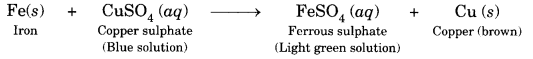Activity 1.10 (Textbook Page 11)

Observation: A white solid, insoluble in water is formed. It is called precipitate.
Conclusion: Barium chloride (BaCl2) reacts with sodium sulphate(Na2SO4) to form a white precipitate of barium sulphate along with sodium chloride in the solution.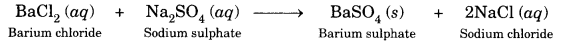Activity 1.11 (Textbook Page 12)

Observation: The brown copper powder turns black.
Conclusion: Copper on heating combines with oxygen to form black copper (II) oxide.